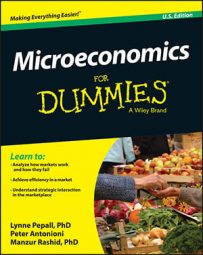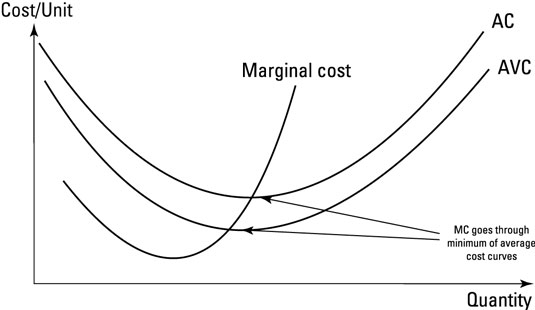##### Microeconomics For DummiesThe breakdowns used for average costs and total costs show costs for a particular level of production. But economists also use another important measure to consider when considering a firm's costs: marginal cost.

## Meeting marginal cost

Put simply, marginal cost (MC) is the cost of adding one extra unit of output to your current output level. (A unit of output could be a ton of steel, a cake, a bushel of wheat, an hour of dental cleaning services, all depending on the output and the units of measurement.) Imagine that you produce 10 beach balls for \$10 in total cost. If you add an extra beach ball to your production and make the total cost \$11, the marginal cost of the 11th beach ball is \$1, because that's how much producing an extra beach ball adds to your total costs.

Economists express marginal costs in terms of the change in total costs, which means that they measure a change in total cost for a change in quantity. Thus marginal costs are a measure not of how much something costs but how much those costs are changing as you do something to production.

"Marginal anything" in economics is important, because it is often the case that decision-making occurs at the margin. If you are already doing something, say making 100 units, and have already made that decision, then you must decide whether to make the 101st unit. That's the marginal decision you face.

You can calculate MC fairly simply, even across a number of units by remembering that it's the change in cost for a small change in quantity (Q). So, quite simply taking the change in total cost (TC) and dividing by the change in quantity gives you an answer:

MC = (Change in TC) / (Change in Q)

## Tracking where MC crosses the AVC and ATC curves

Marginal cost curves always cross the average variable and average total cost curves at the minimum of those curves — that is, at the bottom of the U-shapes that make up both curves. (Average fixed costs are different: they can fall over the whole of the production range and therefore not have a bottom of the U). This intersection has to happen because marginal costs capture the change in costs that determines whether or not average variable or average total costs are rising or falling.

To see why, move away from costs and think about worms for a while. Suppose a biologist is working out the average length of wiggly worms in a sample. Suppose she's measured 10 of them and come up with an average length of 5 inches. Now imagine that she's given an 11th sample. If the 11th worm is longer than 5 inches, the average length of the worms in her sample goes up. If it's less than 5 inches, of course, the average in the sample goes down. But if the marginal worm is exactly 5 inches long, the average stays at exactly 5 inches.

Back to costs. Suppose the average cost of producing ten units is \$1. If the cost of adding an extra unit is greater than \$1, the total cost of producing all the units is more than \$11, and the average cost of production is more than \$1. But suppose that the marginal cost of an extra unit is 90 cents. Then the total cost is \$10.90 and average cost is 99 cents — average cost has fallen. On the other hand, if the marginal cost is exactly \$1, average cost is also exactly \$1.

So you have three cases:

• Average cost falls when the marginal cost of producing an extra unit is less than the average cost of producing all previous units.

• Average cost rises when the marginal cost of the extra unit is greater than the average cost of producing all preceding units.

• Average cost stays the same when the marginal cost of producing an extra unit is exactly the same as the average cost of producing the previous units.

You can see this effect illustrated graphically in the following figure. These "typical" average curves are split at their minimum points (bottom of the U) by a marginal cost curve.Relationship between average (AC) and marginal cost (MC). AVC = average variable costs.

Here's a key implication. To minimize costs, you want to be at the minimum of the average total cost curve (i.e., the minimum of AC). You're at that point when the marginal cost of adding an extra unit of production is exactly the same as the average costs of all the preceding units.

So, to produce for the lowest possible cost, you know not to produce when the marginal cost is greater than average cost, and that if your marginal cost curve is below average cost, you're better off in terms of average cost when you expand your production up to the point where they're equal.